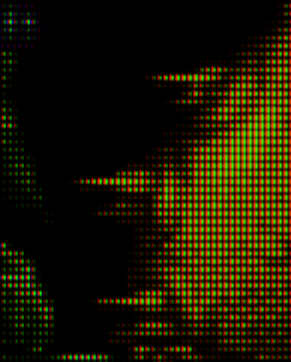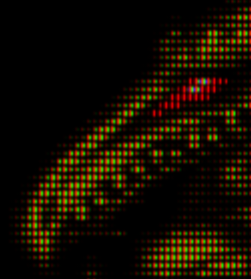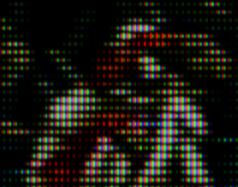# Please show off what crt shaders can do!

Playing around with a new filter for the ntsc-fast version:

7 Likes

Is this filter to create the rainbow effect?

1 Like

Hey everyone quick update, I have updated my 8/16 bit shaders from my last shader pack post. There was excessive jittering going on and I managed to not only reduce it but also sharpen up the overall picture a little more in the process. That juicy rainbow banding now looks better than ever. You can grab the new dl link at the same post here.

4 Likes

If you use the latest version of the ntsc shaders, then resolution scaling can be combined with sharpening to create different coloring effects. It’s also important if the ntsc shaders operate with 2 or 3 phase filtering. Anything above 300 horizontal original resolution triggers 2-phase by default.

It’s also possible that different horizontal resolutions produce a different effect in some situations:

If i play with resolution scaling a bit i get this:

An interesting remark about photos of the rainbow effect would be, that 2-phase encoding/decoding produce different odd/even frames, probably on real HW too. It’s also especially hard to grasp the 2-phase screenies, because merging the frames produces color artifact free images and normal screenshots only an odd or even frame. So to speak TV photos might not consider temporal merging.

I’m also demonstrating a new pixel filter with the ntsc-fast version, which can produce a much smoother image if set accordingly.

TL;DR;

With newest versions of the ntsc shaders resolution scaling and sharpening can be used to adjust the ‘rainbow’ effect.

9 Likes

Here we go again another guest shader update another shader pack update. First notable change about this pack is I gave arcade systems its own shader presets so now we have preset shaders for 8/16 bit, 32/64/128 bit and now one for arcade systems bringing the shader pack up to 18 presets for you to play with:

All 8/16 bit game pics were taken with “NTSC Signal Mode” in the parameters turned off (except the Sonic pic, had to show off that rainbow banding). Now you can switch between the RF cable look or a crispy clean sharp look on the fly simply by going into parameters and turning off/on the very first setting.

You must have guest’s latest shader update for my presets to display properly which you can find here.

@HyperspaceMadness this new set will replace the last one. As always, hope you all enjoy.

Edit: As of 06/01 I went and refined the sharpness a bit on all presets so if you downloaded the pack before please grab it again.

8 Likes

maybe this is useful for you bud. Thanks for sharing as always. Now you can control the rainbow intensity. @sonkun

``````shaders = "18"
filter_linear0 = "false"
wrap_mode0 = "clamp_to_border"
mipmap_input0 = "false"
alias0 = ""
float_framebuffer0 = "false"
srgb_framebuffer0 = "false"
filter_linear1 = "true"
wrap_mode1 = "clamp_to_border"
mipmap_input1 = "false"
alias1 = "StockPass"
float_framebuffer1 = "false"
srgb_framebuffer1 = "false"
scale_type_x1 = "source"
scale_x1 = "1.000000"
scale_type_y1 = "source"
scale_y1 = "1.000000"
filter_linear2 = "true"
wrap_mode2 = "clamp_to_border"
mipmap_input2 = "false"
alias2 = "AfterglowPass"
float_framebuffer2 = "false"
srgb_framebuffer2 = "false"
scale_type_x2 = "source"
scale_x2 = "1.000000"
scale_type_y2 = "source"
scale_y2 = "1.000000"
filter_linear3 = "true"
wrap_mode3 = "clamp_to_border"
mipmap_input3 = "false"
alias3 = "PrePass0"
float_framebuffer3 = "false"
srgb_framebuffer3 = "false"
scale_type_x3 = "source"
scale_x3 = "1.000000"
scale_type_y3 = "source"
scale_y3 = "1.000000"
filter_linear4 = "false"
wrap_mode4 = "clamp_to_border"
mipmap_input4 = "false"
alias4 = "NPass1"
float_framebuffer4 = "true"
srgb_framebuffer4 = "false"
scale_type_x4 = "source"
scale_x4 = "4.000000"
scale_type_y4 = "source"
scale_y4 = "1.000000"
filter_linear5 = "true"
wrap_mode5 = "clamp_to_border"
mipmap_input5 = "false"
alias5 = ""
float_framebuffer5 = "true"
srgb_framebuffer5 = "false"
scale_type_x5 = "source"
scale_x5 = "0.500000"
scale_type_y5 = "source"
scale_y5 = "1.000000"
filter_linear6 = "true"
wrap_mode6 = "clamp_to_border"
mipmap_input6 = "false"
alias6 = ""
float_framebuffer6 = "false"
srgb_framebuffer6 = "false"
scale_type_x6 = "source"
scale_x6 = "1.000000"
scale_type_y6 = "source"
scale_y6 = "1.000000"
filter_linear7 = "true"
wrap_mode7 = "clamp_to_border"
mipmap_input7 = "false"
alias7 = "NtscPass"
float_framebuffer7 = "false"
srgb_framebuffer7 = "false"
scale_type_x7 = "source"
scale_x7 = "1.000000"
scale_type_y7 = "source"
scale_y7 = "1.000000"
filter_linear8 = "true"
wrap_mode8 = "clamp_to_border"
mipmap_input8 = "true"
alias8 = "PrePass"
float_framebuffer8 = "false"
srgb_framebuffer8 = "false"
scale_type_x8 = "source"
scale_x8 = "1.000000"
scale_type_y8 = "source"
scale_y8 = "1.000000"
filter_linear9 = "true"
wrap_mode9 = "clamp_to_border"
mipmap_input9 = "true"
alias9 = "AvgLumPass"
float_framebuffer9 = "false"
srgb_framebuffer9 = "false"
scale_type_x9 = "source"
scale_x9 = "1.000000"
scale_type_y9 = "source"
scale_y9 = "1.000000"
filter_linear10 = "true"
wrap_mode10 = "clamp_to_border"
mipmap_input10 = "false"
alias10 = "LinearizePass"
float_framebuffer10 = "true"
srgb_framebuffer10 = "false"
scale_type_x10 = "source"
scale_x10 = "1.000000"
scale_type_y10 = "source"
scale_y10 = "1.000000"
filter_linear11 = "true"
wrap_mode11 = "clamp_to_border"
mipmap_input11 = "false"
alias11 = "Pass1"
float_framebuffer11 = "true"
srgb_framebuffer11 = "false"
scale_type_x11 = "viewport"
scale_x11 = "1.000000"
scale_type_y11 = "source"
scale_y11 = "1.000000"
filter_linear12 = "true"
wrap_mode12 = "clamp_to_border"
mipmap_input12 = "false"
alias12 = ""
float_framebuffer12 = "true"
srgb_framebuffer12 = "false"
scale_type_x12 = "absolute"
scale_x12 = "640"
scale_type_y12 = "source"
scale_y12 = "1.000000"
filter_linear13 = "true"
wrap_mode13 = "clamp_to_border"
mipmap_input13 = "false"
alias13 = "GlowPass"
float_framebuffer13 = "true"
srgb_framebuffer13 = "false"
scale_type_x13 = "absolute"
scale_x13 = "640"
scale_type_y13 = "absolute"
scale_y13 = "480"
filter_linear14 = "true"
wrap_mode14 = "clamp_to_border"
mipmap_input14 = "false"
alias14 = ""
float_framebuffer14 = "true"
srgb_framebuffer14 = "false"
scale_type_x14 = "absolute"
scale_x14 = "640"
scale_type_y14 = "absolute"
scale_y14 = "480"
filter_linear15 = "true"
wrap_mode15 = "clamp_to_border"
mipmap_input15 = "false"
alias15 = "BloomPass"
float_framebuffer15 = "true"
srgb_framebuffer15 = "false"
scale_type_x15 = "absolute"
scale_x15 = "640"
scale_type_y15 = "absolute"
scale_y15 = "480"
filter_linear16 = "true"
wrap_mode16 = "clamp_to_border"
mipmap_input16 = "false"
alias16 = ""
float_framebuffer16 = "true"
srgb_framebuffer16 = "false"
scale_type_x16 = "viewport"
scale_x16 = "1.000000"
scale_type_y16 = "viewport"
scale_y16 = "1.000000"
filter_linear17 = "true"
wrap_mode17 = "clamp_to_border"
mipmap_input17 = "false"
alias17 = ""
float_framebuffer17 = "false"
srgb_framebuffer17 = "false"
scale_type_x17 = "viewport"
scale_x17 = "1.000000"
scale_type_y17 = "viewport"
scale_y17 = "1.000000"
TVOUT_RESOLUTION = "320.000000"
TVOUT_TV_COLOR_LEVELS = "1.000000"
TVOUT_RESOLUTION_I = "184.000000"
TVOUT_RESOLUTION_Q = "105.599998"
ntscsignal = "1.000000"
avalue = "0.000000"
bvalue = "0.000000"
scantime = "51.800011"
signaloffset = "0.000000"
CS = "1.000000"
TNTC = "4.000000"
ntsc_sat = "0.900000"
ntsc_bright = "0.800000"
SHARPEN = "4.000000"
CONTR = "0.000000"
SIGMA_HOR = "2.699999"
glow = "0.200000"
bloom = "0.250000"
bloom_dist = "0.700000"
brightboost = "1.650000"
textures = "SamplerLUT1;SamplerLUT2;SamplerLUT3;SamplerLUT4"
SamplerLUT1_linear = "true"
SamplerLUT1_wrap_mode = "clamp_to_border"
SamplerLUT1_mipmap = "false"
SamplerLUT2_linear = "true"
SamplerLUT2_wrap_mode = "clamp_to_border"
SamplerLUT2_mipmap = "false"
SamplerLUT3_linear = "true"
SamplerLUT3_wrap_mode = "clamp_to_border"
SamplerLUT3_mipmap = "false"
SamplerLUT4_linear = "true"
SamplerLUT4_wrap_mode = "clamp_to_border"
SamplerLUT4_mipmap = "false"``````
5 Likes

That’s nice. The first thing I noticed is that you turned the signal offset setting off so that the rainbows appear even when you’re not moving around and you’re welcome. These presets should hold out for a bit unless guest bring in some more goodies to add into the shader

3 Likes

CyberLab Slot Mask for Blargg + CyberLab Genesis Blargg S-Video FilterCyberLab Slot Mask for Blargg + CyberLab Genesis Blargg Composite FilterYou have to load full resolution, then open in new tab or window, zoom in or view fullscreen in order for these to look correct because of the mask and scanline settings.

2 Likes

Hey everyone, I went and tweaked the sharpness a bit on all my presets, it’s subtle but I wanted to make things a little sharper. Here’s how it looked before:

Here’s how it looks now:

You can grab the new pack in the same post as before right here.

9 Likes

@HyperspaceMadness do you (or anyone else) still have my ReShade VHS setup? My backup drive died and I replaced the one in my google drive with a newer version.

5 Likes

Take a look at these amazing CRT-Guest-Advanced scanline dynamics!7 Likes

You’re in luck, I’ve got this one:

https://1drv.ms/u/s!AlJgyN_LYasy1JUOYnDJmiLKj_rufw?e=g9BfdH

6 Likes

Updated VGA Monitor preset. For 1440p displays and higher. Using `crt-guest-advanced-hd.slangp`. Shader parameters:

``````pre_bb = "1.020000"
GAMMA_INPUT = "1.750000"
interm = "0.000000"
S_SHARP = "1.500000"
HSHARP = "2.000000"
bloom = "0.100000"
bloom_dist = "0.500000"
halation = "0.050000"
gamma_c = "0.960000"
scangamma = "3.5"
mcut = "0.800000"
mshift = "1.000000"
mclip = "0.350000"
deconrr = "0.500000"
deconrg = "0.250000"
deconrb = "-0.250000"
deconrry = "-0.250000"
deconrgy = "-0.500000"
deconrby = "0.250000"
noiseresd = "1.000000"
noisetype = "1.000000"
post_br = "1.050000"
``````
4 Likes

this is loaded from exe file. Is there some way to load it from RetroArch like a preset ? Thanks for sharing bud.

1 Like

If this is your first time using ReShade. All you have to do is copy and paste the following files and folder to the root of your RetroArch folder (where the retroarch.exe is located) :

ReShade-VHS (rename this folder to just ReShade)

ReShade64.dll (rename this to the video driver you are using in RetroArch. Like to OpenGL32.dll for GL and GLCORE or to DXGI.dll for D3D10/11/12)

That is all. Hope you like it.

P.S. - If you are using Vulkan in RetroArch (or any other game) you can learn how to install ReShade for Vulkan here - https://reshade.me

6 Likes

Hi!

Could you please give me a hand / point me in the right direction to get this / such shader working in DOSBox? I use DOSBox staging and tried a few shaders from dosbox-svn-shaders-master.zip. They work but seems most are for the older EGA 15 KHz monitor look with fat scanlines, but I’m looking for a shader for the double scanned 31 KHz look like in your screenshots.

Thank you!

1 Like

This shader is not available for stand-alone dosbox. You can try hyllian (I think that’s available.) Make sure you configure dosbox to `vgaonly`. If you need SVGA, then make sure to use the 2x scaler if the game runs at 320x200 or 320x240.

Or, just use DOSBox-core in retroarch. Then you can use this exact preset I posted using the crt-guest-advanced shader.

2 Likes

OK, I just tested dosbox-svn with:

``````[sdl]
fullresolution = desktop
windowresolution = 1440x1080
output = openglnb

[dosbox]
machine  = vgaonly

[render]
aspect    = true
scaler    = none